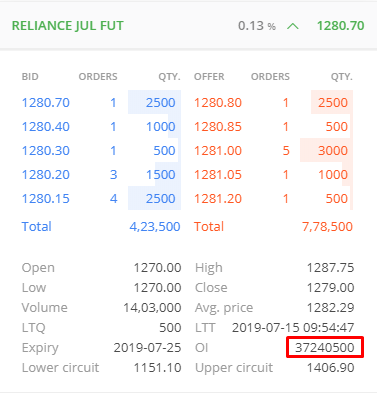# Open interest shown in contracts

@siva I want to know that o.i. given in a scrip is actual o.i. or number of contracts multiplied by lot size

Number of contracts

No number of shares

One long and one position forms one contract, so in FnO terminologies it is number of contracts (as far as I remember)

OI displayed on Kite is the number of contracts multiplied by the lot size.
For example,OI of 3,72,40,500 in Reliance July Futures would mean there are 74481(37240500/500) lots of open contracts.